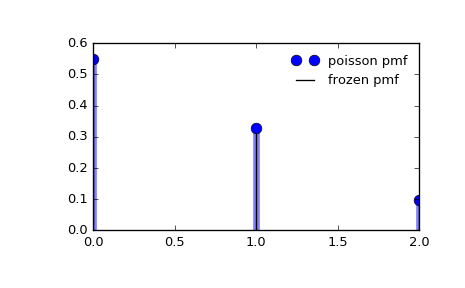# scipy.stats.poisson¶

scipy.stats.poisson = <scipy.stats._discrete_distns.poisson_gen object at 0x2b2318ef6750>[source]

A Poisson discrete random variable.

As an instance of the rv_discrete class, poisson object inherits from it a collection of generic methods (see below for the full list), and completes them with details specific for this particular distribution.

Notes

The probability mass function for poisson is:

poisson.pmf(k) = exp(-mu) * mu**k / k!


for k >= 0.

poisson takes mu as shape parameter.

The probability mass function above is defined in the “standardized” form. To shift distribution use the loc parameter. Specifically, poisson.pmf(k, mu, loc) is identically equivalent to poisson.pmf(k - loc, mu).

Examples

>>> from scipy.stats import poisson
>>> import matplotlib.pyplot as plt
>>> fig, ax = plt.subplots(1, 1)


Calculate a few first moments:

>>> mu = 0.6
>>> mean, var, skew, kurt = poisson.stats(mu, moments='mvsk')


Display the probability mass function (pmf):

>>> x = np.arange(poisson.ppf(0.01, mu),
...               poisson.ppf(0.99, mu))
>>> ax.plot(x, poisson.pmf(x, mu), 'bo', ms=8, label='poisson pmf')
>>> ax.vlines(x, 0, poisson.pmf(x, mu), colors='b', lw=5, alpha=0.5)


Alternatively, the distribution object can be called (as a function) to fix the shape and location. This returns a “frozen” RV object holding the given parameters fixed.

Freeze the distribution and display the frozen pmf:

>>> rv = poisson(mu)
>>> ax.vlines(x, 0, rv.pmf(x), colors='k', linestyles='-', lw=1,
...         label='frozen pmf')
>>> ax.legend(loc='best', frameon=False)
>>> plt.show()Check accuracy of cdf and ppf:

>>> prob = poisson.cdf(x, mu)
>>> np.allclose(x, poisson.ppf(prob, mu))
True


Generate random numbers:

>>> r = poisson.rvs(mu, size=1000)


Methods

 rvs(mu, loc=0, size=1, random_state=None) Random variates. pmf(k, mu, loc=0) Probability mass function. logpmf(k, mu, loc=0) Log of the probability mass function. cdf(k, mu, loc=0) Cumulative distribution function. logcdf(k, mu, loc=0) Log of the cumulative distribution function. sf(k, mu, loc=0) Survival function (also defined as 1 - cdf, but sf is sometimes more accurate). logsf(k, mu, loc=0) Log of the survival function. ppf(q, mu, loc=0) Percent point function (inverse of cdf — percentiles). isf(q, mu, loc=0) Inverse survival function (inverse of sf). stats(mu, loc=0, moments='mv') Mean(‘m’), variance(‘v’), skew(‘s’), and/or kurtosis(‘k’). entropy(mu, loc=0) (Differential) entropy of the RV. expect(func, args=(mu,), loc=0, lb=None, ub=None, conditional=False) Expected value of a function (of one argument) with respect to the distribution. median(mu, loc=0) Median of the distribution. mean(mu, loc=0) Mean of the distribution. var(mu, loc=0) Variance of the distribution. std(mu, loc=0) Standard deviation of the distribution. interval(alpha, mu, loc=0) Endpoints of the range that contains alpha percent of the distribution

#### Previous topic

scipy.stats.planck

#### Next topic

scipy.stats.randint